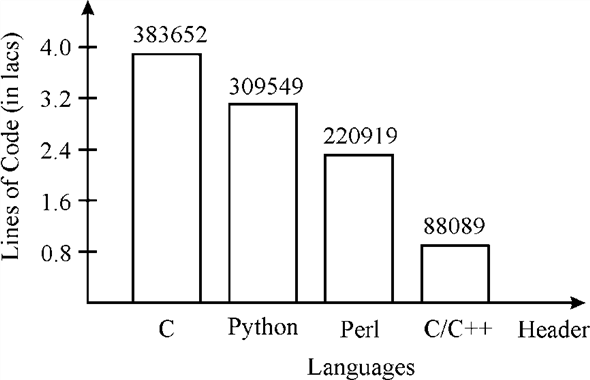# Quiz 12: Computer Programming

Computing

Problem statement It is a set of elements which are manipulated to get a desired result. Each problem statement has following features: • It must depict necessary assumption which is necessary to determine the problem scope. • It clearly depicts the information which is already known. • The duration required to solves a problem. An assumption is something which is accepted as true in order to solve a problem like assumption in a living room floor problem which is rectangular. Known information is the information or input taken from the user about problem which is required in problem solving like living room floor problem in order to calculate the area of pizza, length and width is required. Therefore, the appropriate words to fill in the blanks are assumption , known and input.

Number of lines of code in windows 7 Windows 7 contains more than 50 million of lines of codes. Windows XP also contains approximately 40 millions of lines of codes. Number of lines of code has no importance. For example a user writes a program with 2000 lines of codes that is good but re-writing the code by using only 1000 lines of codes may give a better output. Programs can be written in any language and library. So the number of lines of codes can vary. It depends upon the language used. A line of code is nothing but instructions for writing a computer program. A line of code is used for measuring the volume of the code. It is only used for comparing two products which are formed by using same type of library and language. A line of code is also used for measuring the complexity of the program. More will be the line of code, complex will be program Consider lines of codes of four languages, C, Perl, Python and C/C++ Header, a ballpark figure is shown below:Algorithm Set of statements or steps in natural language to perform any specific task is called as algorithm like dijkstra algorithm to find the shortest path in graph. Set of instruction in any programing language like in C++, C# to perform any specific task is called as program. The implantation of algorithm is called as program. A program takes inputs from user then gives the result as per the inserted input. Like in living room floor program takes width and length from user and then after calculation display area. Therefore, the appropriate words to fill in the blank are algorithm and program.